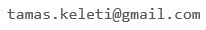﻿ BSM

## Conjecture and Proof

• Instructor: Tamas Keleti
• Contact:• Prerequisites: Introductory math courses, strong problem solving skills
• Text: M. Laczkovich: Conjecture and Proof, Typotex, 1998 (avaliable in digital format, too) and handouts

Description: We try to follow the instruction given by Paul Erdős: Conjecture and prove! According to his spirit, the course intends to show the many and often surprising interrelations between the various branches of mathematics (algebra, analysis, combinatorics, geometry, number theory and set theory) and also to exhibit several unexpected mathematical phenomena via the active problem solving of the students.

Among others we plan to find answers to the following questions:

• Can the real function f(x)=x be written as the sum of (finitely many) periodic functions?
• Is there a power of 2 whose first 2021 digits are all 7?
• Can you "cut" a ball into finitely many (not necessarily "nice") subsets and "reassemble" two(!) copies of the original ball?
• Can you tile a rectangle of size 1 x √2 with squares?
• Can you construct two subsets of the plane so that their union is congruent (in the usual geometric sense) to both subsets?

The main activity in our meetings is not lecturing. You will need to present your solutions to the assigned problems and to understand, check and discuss solutions of other students. To present your solutions you will need to have a drawing tablet or a normal tablet with a stylus.

A large part of the problems are pretty challenging, this is why strong problem solving skills are needed. The main goal of the course is not to improve but to use your already existing skills to reach deep, beautiful and surprising phenomena via problem solving.

Course Topics:

Invariants for proving impossibility, applications in combinatorics and number theory

Irrational, algebraic and transcendental numbers, their relations to approximation by rationals

Vector spaces, Hamel bases, Cauchy's functional equation, applications

Countable sets, cardinalities, applications of Axiom of Choice and Zorn's Lemma

Isometries of the plane and the space

Isometries, geometric and paradoxical decompositions: Bolyai--Gerwien theorem, Hilbert's third problem, Banach--Tarski paradox

Finite and infinite games, winning strategies